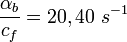# Difference between revisions of "Exercise: Moving your finger through a flame without getting burned"

(Contributed by Demetri Spanos, 3 Oct 04)

In this problem we will look at how to play with fire without getting burned. The system we want to consider is a finger being moved back and forth across a flame, as shown below: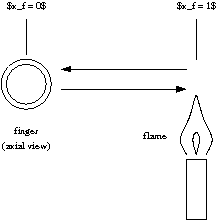The description of the system is as follows:

Using the MATLAB {\tt ode45} function (or something similar), build a model for the system and solve the following:

1. Assume that the finger moves sinusoidally in and out of the flame at frequency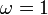rad/sec. Plot the temperature of the finger as a function of time and identify the transient and steady state response.

2. Plot the {\em steady state} amplitude of the finger temperature as a function of the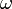forranging from 1 to 100 rad/sec. You should get something similar to the frequency response plot shown in lecture on Monday. You should compute at least 5 points in your graph.

3. Double the gain of the temperature control system by increasing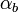by a factor of 2. Replot the frequency response from part~b and describe in words how it differs from the original gain (i.e., where is the response bigger, smaller or unchanged and what is the reason).

You should use the following parameter values in your simulations:

•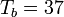,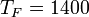degrees Celsius.

•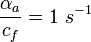•